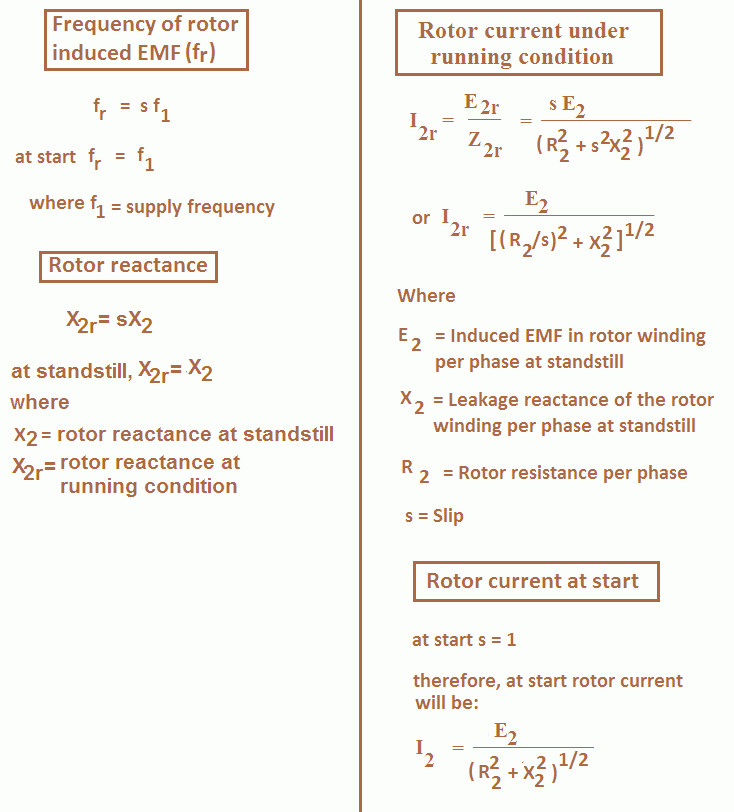# Slip of Induction Motor

Hi friends, in this article I am describing the importance of slip in induction motor and hoping you will find it informative and useful.

In the induction motor rotor always rotates speed less than synchronous speed. The difference between the rotor speed (N) and the rotating magnetic flux speed (Ns) is called slip. The induction motor slip is usually expressed as a percentage of synchronous speed (Ns) and is represented by symbol s.

Mathematically, Percentage slip, % s = [(Ns – N)/Ns] x 100

or Fractional slip, s = (Ns – N)/Ns

The difference between synchronous speed and rotor speed is called slip speed
i.e. Slip speed = Ns – N

The value of slip in induction motor at full load varies from about 6% for small motors to about 2% for large motors.

# Significance of Slip in Induction Motor

Slip of induction motor plays an important role in the operation of the induction motor. The torque produced by the induction motor is directly proportional to induction motor slip. At no-load induction motor requires small torque to meet with the frictional, iron and other losses, therefore slip is small.

When the motor is loaded, greater torque is required to drive the load, therefore, slip increases and rotor speed decreases slightly. Thus induction motor slip adjusts itself to such a value so as to meet the required driving torque.

At standstill N = 0 hence s = 1 whereas at N = Ns, s = 0 (imaginary condition).

## Induced EMF in Rotor

E2r = sE2

Where E2 = Induced EMF per phase at standstill.
E2r = Rotor induced EMF per phase in running condition.

At standstill s = 1 hence E2r = E2 whereas at N = Ns, s = 0, E2r = 0.

Thus rotor induced EMF fluctuates between 0 and E2 for the rotor speeds between N = Ns and N = 0.

## Frequency of Induced EMF in Rotor

The expression for frequency of induced EMF in the rotor is:

fr = sf1

i.e. rotor EMF frequency = fractional slip x supply frequency

At standstill, induction motor slip s is 1, hence the frequency of induced EMF in the rotor of the induction motor is same as that of supply frequency and reduces with increase in speed (due to the reduction in slip).

## Rotor Resistance

There is no effect of rotor induced EMF frequency on its resistance. Hence the rotor resistance remains constant irrespective of the speed of the induction motor.

## Rotor Reactance

Let X2 be the rotor reactance per phase at standstill. The rotor frequency at standstill is fr = f1.

Therefore, X2 = 2πf1L2 ohm/phase

In the running condition the frequency of rotor voltage is fr = sf1. Hence rotor reactance in the running condition X2r is given by,

X2r = 2πfrL2 = 2πsf1L2 = s (2πf1L2) = sX2

X2r = sX2.

At standstill, induction motor slip s is 1, hence reactance of rotor of the induction motor is same as reactance of rotor at standstill and reduces with increase in speed (due to the reduction in slip).## Rotor Impedance

The rotor impedance per phase at standstill is given by,

Z2 = (R22 + X22)1/2 ohm/phase

The rotor impedance per phase at running condition is given by,

Z2r = (R22 + sX22)1/2 ohm/phase

Where R2 = Rotor resistance per phase
X2 = Reactance of the rotor winding per phase at standstill.

## Rotor Power Factor

At standstill, the rotor power factor is given by,

cos φr = R2/Z2 = R2/(R22 + X22)1/2

At running condition, the rotor power factor is given by,

cos φ2r = R2/Z2r = R2/[R22 + (sX2)2]1/2

Thanks for reading about significance of slip in induction motor.

## Three Phase Induction Motor | All Posts

© //www.yourelectricalguide.com/ significance of slip in induction motor.

### 1 thought on “Slip of Induction Motor”

1.Type here.. good notes